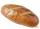Mr. John

Mr. John wants to buy winter apples Spartan. There are two ways to buy:

1) buy apples at the marketplace, where 1 kg costs 15 CZK.
2) Bring the car to the agricultural farm, where the apples are sold after 9 CZK per kilogram. However, he must pay CZK 150 for car gasoline.

How many kilograms of apples would have Mr. John buy that for him to be more cost-effective to drive to the agricultural farm?

Result

x =  25 kg

Solution:

15x = 9x+150

6x = 150

x = 25

Calculated by our simple equation calculator.

Leave us a comment of this math problem and its solution (i.e. if it is still somewhat unclear...):Be the first to comment!To solve this verbal math problem are needed these knowledge from mathematics:

Do you have a linear equation or system of equations and looking for its solution? Or do you have quadratic equation?

Next similar math problems:

1. Forest nurseryIn the forest nursery after winter, they found that 1/10 stems died out of them. For them, they land 193 new spruces. How many spruces are in the forest nursery?
2. Simple equation 8Solve the following equation: 36=-(1+7x)-6(-7-x)
3. Negative in equation2x + 3 + 7x = – 24, what is the value of x?
4. Unknown number 11That number increased by three equals three times itself?
5. Unknown numberIdentify unknown number which 1/5 is 40 greater than one tenth of that number.
6. NormThree workers planted 3555 seedlings of tomatoes in one dey. First worked at the standard norm, the second planted 120 seedlings more and the third 135 seedlings more than the first worker. How many seedlings were standard norm?
7. Simple equationSolve the following simple equation: 2. (4x + 3) = 2-5. (1-x)
8. InequationSolve the inequation: 5k - (7k - 1)≤ 2/5 . (5-k)-2
9. One-thirdA one-third of unknown number is equal to five times as great as the difference of the same unknown number and number 28. Determine the unknown number.
10. EquationSolve the equation: 1/2-2/8 = 1/10; Write the result as a decimal number.
11. Simple equationSolve for x: 3(x + 2) = x - 185 of the same bread has the same weight as three bread and 4 kg of fruit. What weight has one bread?Solve the following equation: -8y+5=-9y+9Three consecutive sheets dropped from the book. The sum of the numbers on the pages of the dropped sheets is 273. What number has the last page of the dropped sheets?Solve: if 2(x-1)=14, then x= (solve an equation with one unknown)?Solve next equation: 2 ( 2x + 3 ) = 8 ( 1 - x) -5 ( x -2 )